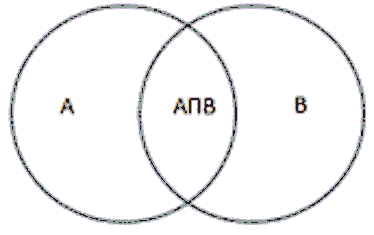# Intersection of Set(A Intersect B) or A∩B Calculator

Calculator will find the Intersection of Set(A Intersect B).

 Enter the values in the set1 (seperated by comma) Enter the values in the set2 (seperated by comma)

 Intersection of sets

#### For example:

The two sets of events A={1,2,3,4} and B={3,4,6,7,8}

the intersection of the sets we get

A ∩ B = {3, 4}

### Definition

Set is the relation of some given data or say set contains the elements of similar type or category. A set is defined as the collection of elements, such as numbers or other objects, which are arranged in a group. The set with any numbers can be denoted in the symbol braces { }. The intersection of the sets A and set B is represented by A ∩ B and it is pronounced as A intersection B.### Differences Between Union and Intersection

Union of SetIntersection of Set
The union discards duplicates values of given sets.An intersection is a set of common values only.
The number of elements of a union is greater than or equal to parent sets.The number of elements in an intersection is always less than or equal to parent sets.
The symbol "∪" is used to represent the union of set.The symbol "∩" is used to represent the intersection of set.